# How to denote ket notation in LaTeX?

Ket notation is like a kind of bracket, one side of which is bound by single bar and the other side by a right angle bracket.

\documentclass{article}
\begin{document}
$| a \rangle$
$\vert \phi \rangle$
$\vert \hat{A} \rangle$
\end{document}

Output :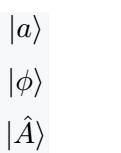Instead of typing such a large syntax, you can define a new command. Latex has multiple methods for defining new commands.

However, in this case, the best practice is to use the mathtools package.

\documentclass{article}
\usepackage{mathtools}
\DeclarePairedDelimiter\ket{\lvert}{\rangle}
\begin{document}
$\ket{\psi}$
$\hat{p}\ket{p}$
$U\ket{a_j}=\ket{Ua_i}$
$\ket{1},\ket{2},\ldots,\ket{k}$
\end{document}

Output :If you use the star * symbol with to this new command. The size of ket symbol will be adjustable with the argument.

\documentclass{article}
\usepackage{mathtools}
\DeclarePairedDelimiter\ket{\lvert}{\rangle}
\begin{document}
$\ket*{\Omega }$
$\ket*{\Omega^\dagger}$
$\ket*{\frac{\Omega^\dagger}{k}}$
% Passing the Big Command as an optional argument
$\ket[\big]{\psi} \ket[\Big]{\frac{\psi}{k}}$
$\ket[\bigg]{\frac{\psi_i}{n}} \ket[\Bigg]{\frac{\psi_i}{n_k}}$
\end{document}

Output :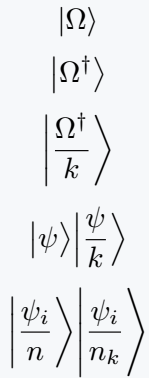## Use braket package for ket notation

This package contains two commands \ket and \Ket. In the case of ket command, size will be fixed. For example

\documentclass{article}
\usepackage{braket}
\begin{document}
$\ket{\psi} = \sum_j \sigma_j\ket{\psi_j}$
$\ket{\phi} = A\ket{\psi_i}$
$\ket{\frac{\psi_i}{k}}$
\end{document}

Output :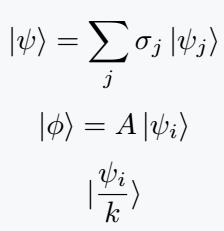And it would be best practice to use the \Ket command because the size of the notation and argument will match dynamically.

\documentclass{article}
\usepackage{braket}
\begin{document}
$\Ket{\frac{m}{k}} = v\Ket{\frac{n}{k}}$
$\Ket{\frac{\psi_i}{k}}$
\end{document}

Output :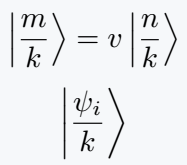\Ket and \ket are considered two separate commands because of latex is case sensitive

## Use physics package in LaTeX

The same command is defined in the physics package as in the bracket package. For example

\documentclass{article}
\usepackage{physics}
\begin{document}
$v \to \ket{v}$
$\ket{v_i} = \sum_{i}\ket{i}\alpha_i$
$\ket{v} = \alpha_1\ket{a_1} + \alpha_2\ket{a_2}$
\end{document}

Output :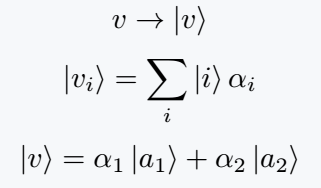Adding a star to the \ket command will make the shape of the notation is constant.

\documentclass{article}
\usepackage{physics}
\begin{document}
$\ket*{v}$
$\ket*{\hat{A}_i}$
$\ket*{\frac{v_i}{k}}$
\end{document}


Output :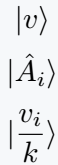#### Md Jidan Mondal

LaTeX expert with over 10 years of experience in document preparation and typesetting. Specializes in creating professional documents, reports, and presentations using LaTeX.